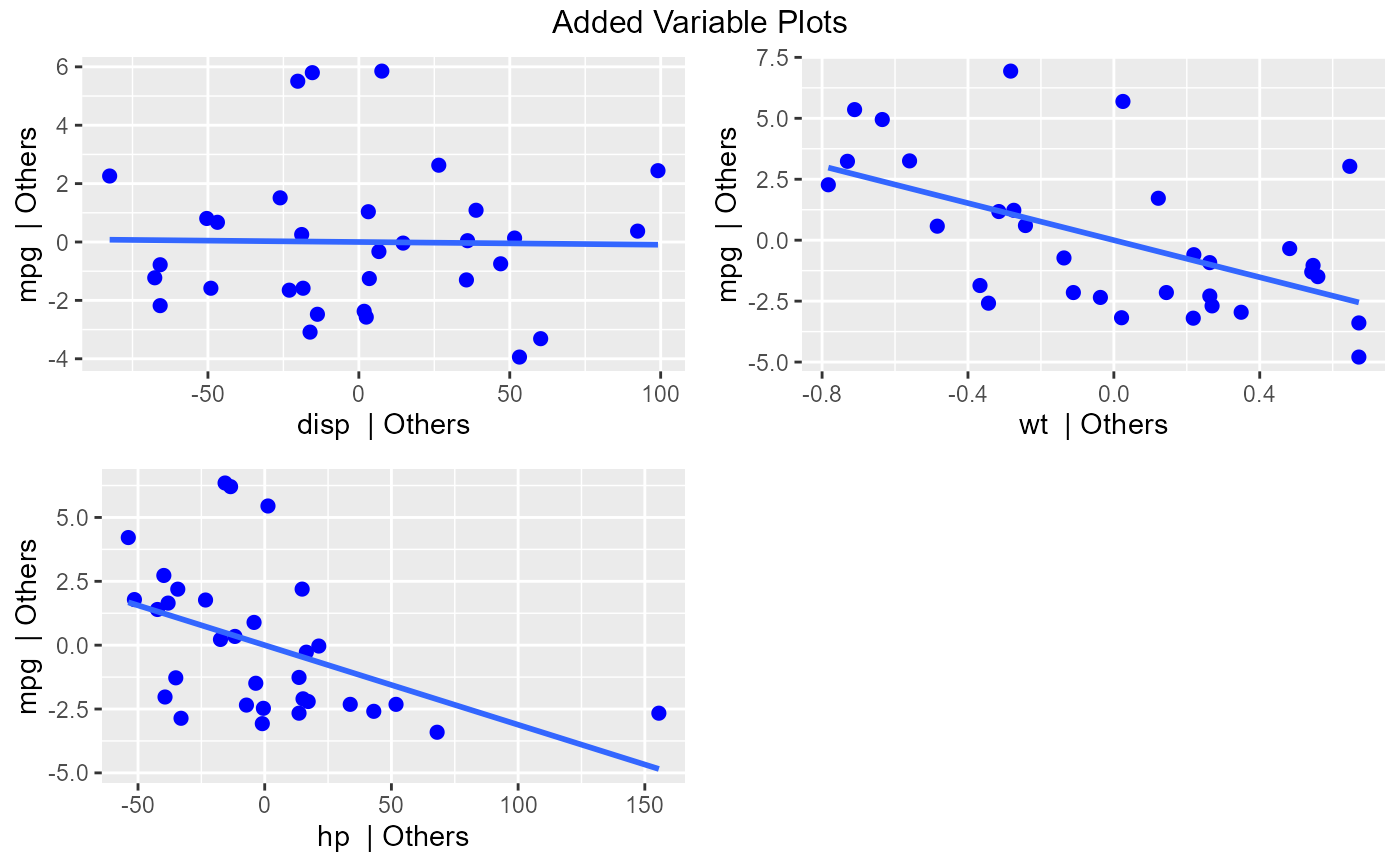Added variable plot provides information about the marginal importance of a predictor variable, given the other predictor variables already in the model. It shows the marginal importance of the variable in reducing the residual variability.

ols_plot_added_variable(model, print_plot = TRUE)

## Arguments

model An object of class lm. logical; if TRUE, prints the plot else returns a plot object.

## Details

The added variable plot was introduced by Mosteller and Tukey (1977). It enables us to visualize the regression coefficient of a new variable being considered to be included in a model. The plot can be constructed for each predictor variable.

Let us assume we want to test the effect of adding/removing variable X from a model. Let the response variable of the model be Y

Steps to construct an added variable plot:

• Regress Y on all variables other than X and store the residuals (Y residuals).

• Regress X on all the other variables included in the model (X residuals).

• Construct a scatter plot of Y residuals and X residuals.

What do the Y and X residuals represent? The Y residuals represent the part of Y not explained by all the variables other than X. The X residuals represent the part of X not explained by other variables. The slope of the line fitted to the points in the added variable plot is equal to the regression coefficient when Y is regressed on all variables including X.

A strong linear relationship in the added variable plot indicates the increased importance of the contribution of X to the model already containing the other predictors.

## Deprecated Function

ols_avplots() has been deprecated. Instead use ols_plot_added_variable().

Chatterjee, Samprit and Hadi, Ali. Regression Analysis by Example. 5th ed. N.p.: John Wiley & Sons, 2012. Print.

Kutner, MH, Nachtscheim CJ, Neter J and Li W., 2004, Applied Linear Statistical Models (5th edition). Chicago, IL., McGraw Hill/Irwin.

model <- lm(mpg ~ disp + hp + wt, data = mtcars)
ols_plot_added_variable(model)